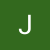# The Blind 75 Leetcode Series: Merge Intervals

Today, we are working on 56. Merge Intervals

Before we start, I would like to add that I’ve seen this problem or something similar to this multiple times throughout my career when I’m interviewing. This is a fun question that really tests your ability to come up with an algorithm that covers all edge cases. Anything that goes along with “meeting room schedule (I got this from the rainforest company)” or “baseball field assignment for little league (I got this from the G)” goes along with this question. A good introductory question that can expand to something more complex, and, rare for Leetcode questions, practical in real life.

Let’s begin.

Given an array of where , merge all overlapping intervals, and return an array of the non-overlapping intervals that cover all the intervals in the input.

I think typical questions to ask before starting to working on this would be

1. Do we expect empty lists?
2. If yes, do we expect only or also as empty list. Do we expect empty list inside a list like
3. How many intervals are we expecting
4. What’s the numbers inside? Does it go very large?
5. Is the array sorted in any way?

If the answers are “No”, “No”, “finite interview, maybe 10⁴”, “also 10⁴”, and “it is not sorted” then we can eliminate some of the cases where numbers > positive 2³² and empty lists.

First, brute force.

We go through each interval, and check against a tracker which contains the combined intervals. The logic is simply: If the interval’s starting point falls within a given tracked interval, we take the max of the tracked end point and the interval’s end point. Ex. tracked = , interval = . falls within and , so we take and use as the new end point, giving as the new tracked interval. If the starting point doesn’t fall into any of the interval, append the interval to tracker.

This approach assumes the new interval starts later than the previous ones to work correctly, so we want to sort the intervals first by the starting points.

`def merge(intervals):    if not intervals:        return []    if len(intervals) == 1:        return intervals    intervals.sort()  # sort by starting points    tracker = [intervals.pop(0)]    while intervals:  # loop through all intervals        interval = intervals.pop(0)        inserted = False        for t in tracker:            if interval >= t and interval <= t:                t = max(t, interval)                inserted = True        if not inserted:  # no existing interval is mutated, append            tracker.append(interval)    return tracker`

What’s the time complexity we are facing?

We first have the sort, which is O(n log n) where n is the length of the intervals. Then we go through each of the interval and for each interval, we go through the each of the intervals in the tracker, so we are looking at O(n²) here.

`def merge(intervals):    if not intervals:        return []    if len(intervals) == 1:        return intervals    intervals.sort()  # O(n log n)    tracker = [intervals.pop(0)]    while intervals:  # O(n)        interval = intervals.pop(0)        inserted = False        for t in tracker:  # O(n)            if interval >= t and interval <= t:                t = max(t, interval)                inserted = True        if not inserted:            tracker.append(interval)    return tracker`

Definitely room for improvement.

Following this brute force approach, we know that the result in tracker should be sorted already because the input intervals are sorted already. If the inputs are , then what’s inside the tracker should be the same. Similarly, with some merged intervals, the intervals inside the tracker should still be sorted. Ex. => .

For a given interval, we only need to compare with the last interval in the tracker to determine if these two need to be merged. If the starting point of the new interval is in-between the last interval in the tracker, we combine. Otherwise, we append it to the tracker directly. The algorithm then becomes

`def merge(intervals):    if not intervals:        return []    if len(intervals) == 1:        return intervals    intervals.sort()    tracker = [intervals.pop(0)]    while intervals:        interval = intervals.pop(0)        if interval > tracker[-1]:  # comparison with last interval in the tracker             tracker.append(interval)        else:            tracker[-1] = max(tracker[-1], interval)    return tracker`

We have eliminated the iteration through the tracker, so now we are only looking at an O(n) for that loop. Combining with the sort, we now have a O(n log n) complexity.

That’s it! Another problem down.